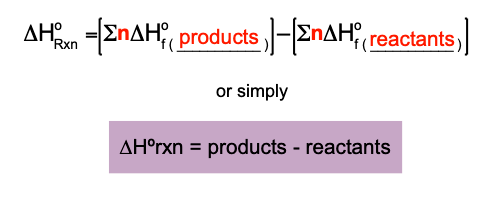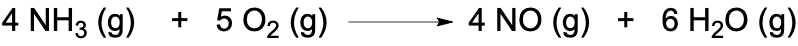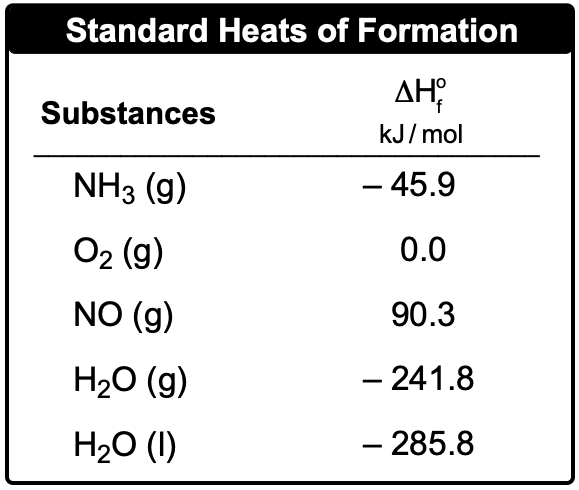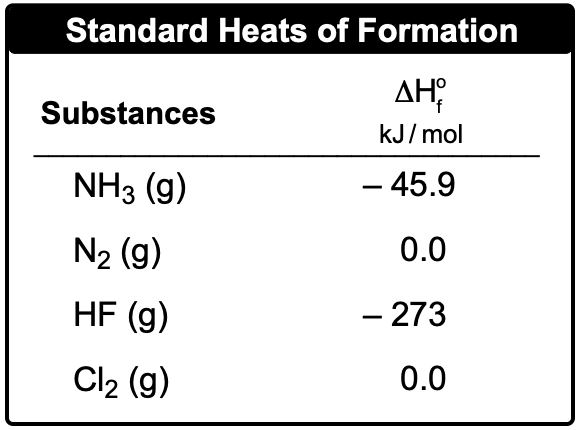Clutch Prep is now a part of Pearson
Ch.6 - Thermochemistry WorksheetSee all chapters

# Enthalpy of Formation

See all sections
Sections
Nature of Energy
Kinetic & Potential Energy
First Law of Thermodynamics
Internal Energy
Endothermic & Exothermic Reactions
Heat Capacity
Constant-Pressure Calorimetry
Constant-Volume Calorimetry
Thermal Equilibrium
Thermochemical Equations
Formation Equations
Enthalpy of Formation
Hess's Law
Enthalpy (IGNORE)

The Enthalpy of Formation for an element is a key component in determining the enthalpy of reaction.

###### Enthalpy of Formation

Concept #1: Enthalpy of FormationExample #1: The reaction of methane with chlorine gas is illustrated by the reaction below:

Calculate the ∆Horxn if the standard enthalpies of formation for CH4 , CCl4 , and HCl are –74.87 kJ/mol, –139 kJ/mol and –92.31 kJ/mol respectively.

Practice: The oxidation of ammonia is illustrated by the following equation:Calculate the enthalpy of reaction, ΔHRxn, based on the given standard heats of formation.Practice: Consider the following equation:

2 ClF3(g) + 2 NH3(g) → 1 N2(g) + 6 HF (g) + 6 Cl2(g)  ΔHrxn = –1196 kJ

Determine the standard enthalpy of formation for chlorine trifluoride, ClF3